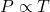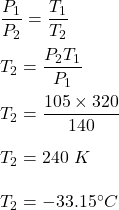## At 47c a gas has a pressure of 140kpa. The gas is cooled until the pressure decreases to 105kpa. If the volume remains constant, what will t

Question

At 47c a gas has a pressure of 140kpa. The gas is cooled until the pressure decreases to 105kpa. If the volume remains constant, what will the final temperature be in kelvin’s? In degrees Celsius

in progress 0
5 months 2021-08-29T01:02:47+00:00 1 Answers 20 views 0

The final temperature is equal to 240 K or -33.15°C

Explanation:

Given that,

Initial temperature of the gas, T₁ = 47°C = 320 K

Initial pressure, P₁ = 140 kpa

Final pressure, P₂ = 105 kpa

We need to find the final temperature if the volume remains constant.  The relation between temperature and pressure is given by :orSo, the final temperature is equal to 240 K or -33.15°C.